Wednesday 5th August 2020# Parents - Educate your children

## This section of the @school web site is to help parents find information and resources to help their children with their education.

•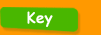•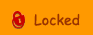•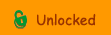••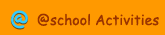•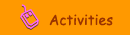•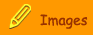###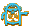Help your Child

Addition  compensation - An introduction to using the compensation method of addition in columns (Year 4-6)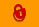Addition  counting on - Learn how to use informal written methods to carry out additions (Year 3)Addition in Columns  Introduction - An introduction to addition in columns. (Year 3)Addition in Columns 2 - An introduction to carrying when adding in columns (Year 4  6).Back Page - Video tips about how to help the kids with Maths and English homework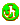Digger and the Gang - Interactive activities that help to guide you through the National Curriculum.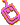Division 1 - An introduction to written methods for division (Year 4)Division 2 - An explanation of written methods for division (Year 5)Edbydesign - Practical ideas and strategies that will give children a positive learning experience.Informal Subtraction  Counting Up - Further progress to using the decomposition method of subtraction in columns (Year 4  6)Long Division - An explanation of written methods for long division (Year 6)Multiplication  grid method - An introduction to the grid method when multiplying two-digit numbers (Year 4 )Multiplication  written methods - An introduction to the partition method when multiplying two-digit numbers (Year 4)Subtraction  compensation - Learn how to use informal written methods to carry out subtractions (Year 3)Subtraction  decomposition - An introduction to using the decomposition method of subtraction in columns (Year 3).Subtraction  decomposition 2 - Further progress with the decomposition method of subtraction in columns (Year 4  6).Word Pool - The childrens book site for parents, teachers and writers.